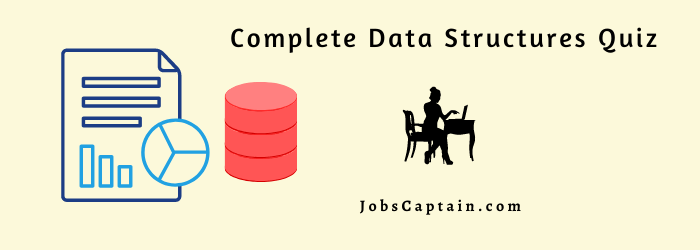# Data Structures Objective Questions and Answers – 4Your welcome to this quick Data Structures Objective Quiz. It has important thirty basic/advance questions about various Data Structures topics.

## Data Structures Objective Questions with Answers

Question 1: Accessing and processing each array elements is called ___________.

(A) sorting

(B) traversing

(C) searching

(D) merging

(B) traversing

Question 2: The sequence (1,1) (2,1) (3,1) (1,2) (2,2) (3,2) . . . . represents __________.

(A) row major order

(B) column major order

(C) random order

(D) successive order

(B) column major order

Question 3: What is not a technique of tree traversal?

(A) pre-order

(B) post-order

(C) prefix

(D) in-order

(C) prefix

Question 4: Selection sort and quick sort both fall into the same category of sorting algorithms. _________ is that category.

(A) O(n log n) sorts

(B) Divide-and-conquer sorts

(C) Interchange sorts

(C) Interchange sorts

Question 5: The possibility of two different keys k1 & k2 yielding the same hash address is called__________.

(A) merge

(B) obstacle

(C) overlapping

(D) collision

(C) overlapping

Question 6: Uniform distribution of the hash address throughout the given set L is _____________.

(A) reduce the number of collision

(B) increase the number of collision

(C) totally avoid collision

(A) reduce the number of collision

Question 7: _________involves maintaining two tables in memory.

(A) Arranging

(B) Bonding

(C) Combing

(D) Chaining

(D) Chaining

Question 8: ___________ is a well defined list of steps for solving a problem.

(A) Algorithm

(B) Program

(C) Procedure

(D) Process

(A) Algorithm

Question 9: The data items in a record form a ________ structure which can be described by means of level numbers.

(A) hierarchical

(B) procedural

(C) indexed

(D) leveled

(A) hierarchical

Question 10: A vertex of degree one is called _____________.

(B) isolated vertex

(C) null vertex

(D) colored vertex

Question 11: A connected graph T without any cycles is called _____________.

(A) a tree graph

(B) free tree

(C) a tree

(D) all of the above

(D) all of the above

Question 12: Which of the following is true while inserting a new node in the list?

(A) Check there is node in the list

(B) Check in the free node in the pool

(C) There is no node

(D) Underflow

(B) Check in the free node in the pool

Question 13: Which of the following data structures are indexed structures?

(A) Linear arrays

(D) Arrays

(A) Linear arrays

Question 14: The efficiency of a BFS algorithm is dependent on _____________ .

(A) Algorithm

(B) Tree

(C) Problem

(D) Graph

(D) Graph

Question 15: Divide and conquer is an important algorithm design paradigm based on ____________.

(A) multi-branched recursion

(B) single-branched recursion

(C) two-way recursion

(D) None

(A) multi-branched recursion

Question 16: The correctness of a divide and conquer algorithm is usually proved by _________.

(A) mathematical theorem

(B) de morgan’s law

(C) mathematical induction

(D) None of above

(C) mathematical induction

Question 17: The ____________ is used in an elegant sorting algorithm.

(A) Heap sort

(B) Quicksort

(C) Merge sort

(A) Heap sort

Question 18: ____________ is finding a path/tour through the graph such that every vertex is visited exactly once.

(A) Travelling Salesman tour

(B) Eulerian tour

(C) Hamiltonian tour

(D) None of above

(C) Hamiltonian tour

Question 19: ____________ data structure is used to implement Depth First search.

(A) Array

(C) Queue

(D) Stack

(D) Stack

Question 20: Each entry in a linked list is a called a_______________.

(B) Node

(C) Data Structure

(D) Avail

(B) Node

Question 21: ____________  is two way lists.

(D) List traversed in two directions

(D) List traversed in two directions

Question 22: In a linked list the _________ field contains the address of next element in the list.

(B) Next element field

(C) Start field

(D) Info field

Question 23: A list that has no nodes is called ____________.

(A) End list

(B) Zero list

(C) Null list

(D) Sentinel list

(C) Null list

Question 24: The special list which consists of unused memory space is called __________.

(A) Free space

(B) Empty space

(C) Available space

(D) Free storage list

(D) Free storage list

Question 25: The efficient searching algorithm for algorithm for a sorted array is _________.

(A) Binary search

(B) Linear search

(C) Indexed search

(D) Repeated search

(A) Binary search

Question 26: To insert a new node in linked list free node will be available in ___________.

(A) Available list

(B) Avail list

(C) Free node list

(D) Memory space list

(B) Avail list

Question 27: A ______________ list is a header list where the node points back to the header node.

Question 28: How many pointers are necessarily changed for the insertion in a Linked List?

(A) 1

(B) 2

(C) 3

(D) 4

(B) 2

Question 29: An algorithm that calls itself directly or indirectly is known as ____________.

(A) Sub algorithm

(B) Recursion

(C) Polish notation

(D) Traversal algorithm

(B) Recursion

Question 30: Which of the following data structures are indexed structures?

(A) Linear arrays